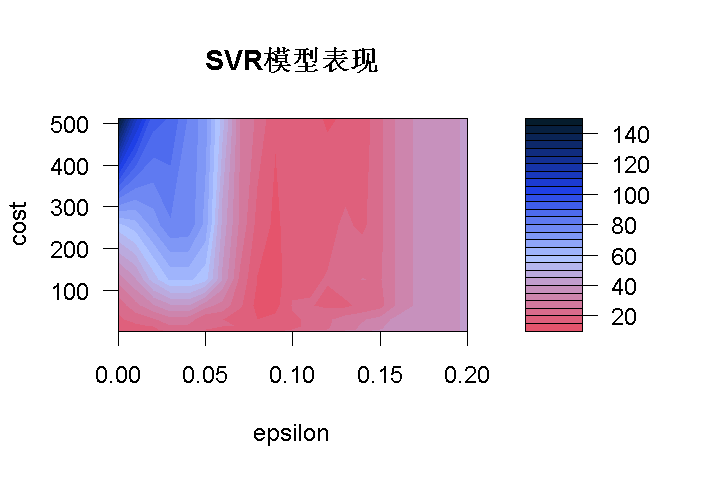# R语言进行支持向量机回归SVR和网格搜索超参数优化

## 第1步：在R中进行简单的线性回归

```# 从csv文件中加载数据

# 绘制数据

plot(data, pch=16)

# 创建一个线性回归模型

model <- lm(Y ~ X, data)

# 添加拟合线

abline(model)```

## 第2步：我们的回归效果怎么样？

```# 对每个X做一个预测

pred <- predict(model, data)

# 显示预测结果

points(X, pred)```

# 使用R，我们可以得到以下代码来计算RMSE

```rmse <- function(error)

{

sqrt(mean(error^2))

}```

## 第3步：支持向量回归

`model <- svm(Y ~ X , data)`

# 这一次的预测结果更接近于真实的数值 ! 让我们计算一下支持向量回归模型的RMSE。

```# 这次svrModel\$residuals与data\$Y - predictedY不一样。

#所以我们这样计算误差

svrPredictionRMSE```

## 第四步：调整你的支持向量回归模型

```# 进行网格搜索

tuneResultranges = list(epsilon = seq(0,1,0.1), cost = 2^(2:9))

# 绘制调参图

plot(Result)```

• 我们使用tune方法训练模型，ϵ=0,0.1,0.2,…,1和cost=22,23,24,…,29这意味着它将训练88个模型（这可能需要很长一段时间
• tuneResult返回MSE，别忘了在与我们之前的模型进行比较之前将其转换为RMSE。

`rangelist(epsilo = seq(0,0.2,0.01), cost = 2^(2:9))`希望对我们来说，我们不必用眼睛去选择最好的模型，R让我们非常容易地得到它，并用来进行预测。

```# 这个值在你的电脑上可能是不同的

# 因为调参方法会随机调整数据

tunedModelRMSE <- rmse(error)```

Kaizong Ye拓端研究室（TRL）的研究员。

​非常感谢您阅读本文，如需帮助请联系我们！QQ在线咨询

15121130882

0571-63341498

## 关注有关新文章的微信公众号

This will close in 0 seconds